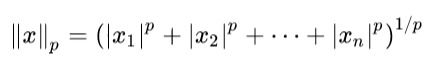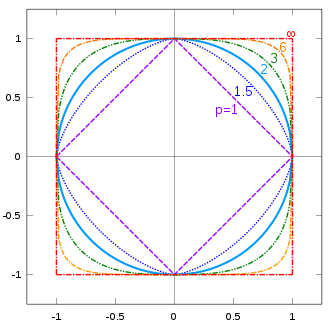top of page
Search
• Divakar V

# H.O.M.L Ch-2 notes | End-to-end ML Project

Updated: Mar 28, 2021

Contents:

In this chapter, the author gives a high level picture of how a machine learning project looks in production phase and tries to highlight some key decisions and choices we come across while developing our machine learning solution.

The experiments were conducted on the California Housing Prices dataset. The goal is to predict housing prices based on various attributes such as location, area population etc.

This post will be more on my key takeaways from the chapter rather than a complete summary explaining the concepts. It was a lengthy chapter exposing several concepts.

## RMSE and MAE

Root mean square error is generally the preferred measure for regression tasks. However, at times, Mean Absolute Error is also used.

RMSE corresponds to l2 norm (Euclidean distance) while MAE corresponds to l1 norm (Manhattan distance)

More generally,l-0 : gives the number of nonzero elements in the vector

l-1 : Manhattan norm

l-2 : Euclidean norm

l-∞ : gives the maximum absolute value in the vector

[Image on right-side: every vector from the origin to the unit circle has a length of one, the length being calculated with length-formula of the corresponding p]

Important Note: The higher the norm index, the more it focuses on large values and neglects the small ones.

Hence, RMSE is more sensitive to outliers that MAE. But when outliers are exponentially rare (like in a bell curve), RMSE performs very well and is generally preferred.

## Few points on test set preparation

When preparing test set, also pay attention to:

• If new data is added or updated, we should have a stable train/test split. This means that the test set should remain consistent across multiple runs and not contain instances which were previously seen in the training set.

• Randomly sampling the test set will only work if the dataset is large enough relative to the number of attributes. Else we might run in sampling bias. Test set should be representative of various attributes of the dataset.

• i.e. take care of Data distribution before sampling and splitting the dataset.

## Correlation• Correlation has nothing to do with Slopes

• The correlation coefficient only measures LINEAR correlations.

## Data Cleaning

Let's say you choose to replace missing values with the Median Value. Take care of 2 things:

• It should only be computed on the Training data

• Save the computed value. It will be required when evaluating the Test set and also to handle missing values when the system goes live.

## Text and Categorical Attributes

Problem with simply converting text to number categories, i.e. converting:

["Apple", "Orange", "Bananas", "Mangoes"] to [0, 1, 2, 3]

is that the algorithm might assume that category "Apple" is closer to "Orange" than it is to "Mangoes". We don't really want this unless categories are something like:Better approach: One-hot-encoding... creating binary attribute per category.

Generally results in sparse matrix of zeroes (image on the right-hand side).

What if categorical attribute has a large number of possible categories?

In that case, one-hot-encoding will result in a big sparse matrix (large number of features). This would mean the training time will increase heavily (slow training) because the algorithm will have to take care a large number of features.

Possible solutions:

1. Replace the categorical input with some other useful numerical feature. E.g. ocean_proximity could be replaced by distance. Country code could be replaced by population and country's GDP

2. Representation Learning - Replace each category with a low-dimensional learnable vector called embeddings.

## Feature Scaling

• ML algorithms do not generally perform well when input attributes have different scales.

• Scaling the target values is generally not required.

### Normalization (min-max scaling)• Values are shifted and scaled to restrict the range between 0 & 1

• Affected by outliers. Say, there is a large value outliers...

### Standardization• Subtract the mean and divide by standard deviation.

• Resulting data has a Unit variance.

• Doesn't restrict the values range.

• Could be problematic for some algorithms which prefers data ranging from 0-1 (e.g. neural networks)

Note... mean & standard deviation to be calculated only on training data.

## Extra notes

1. Scikit-Learn relies on duck typing (not inheritance). It doesn't check the type of the data but the methods it implements.

2. SKlearn's cross-validation expects a utility function (higher the better) rather than a cost function. So, we deal this by adding a minus sign.

3. Use "joblib" library for serializing trained models. More effective than pickle.

4. Just like backing up models, you should also backup your dataset. Will help if the existing one gets corrupted or to evaluate any model against the previous dataset.

5. For deeper understanding of the models' strengths and weaknesses, we can create subsets of test set for specific parts of the data and evaluate across it.

See All
bottom of page